# Chapter 5 Solutions

17,21,27,41,84,86;18,24,32,44,74,92;33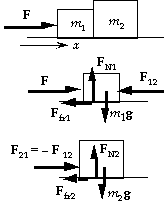```17.	(a)	The two crates must have the same acceleration.
From the force diagram for crate 1 we have
x-component:   F - F12 -  ukFN1 = m1a;
y-component:   FN1 - m1g = 0,  or  FN1 = m1g.
From the force diagram for crate 2 we have
x-component:   F12 - ukFN2 = m2a;
y-component:   FN2 - m2g = 0,  or  FN2 = m2g.
If we add the two x-equations, we get
F - ukm1g -  ukm2g = m1a + m2a;
F - uk(m1 + m2)g  = (m1 + m2)a;
750 N - (0.12)(80 kg + 210 kg)(9.80 m/s2) =
(80 kg + 210 kg)a, which gives a =       1.4 m/s2.
(b)	We can find the force between the crates from the
x-equation for crate 2:
F12 - ukm2g = m2a;
F12 - (0.12)(210 kg)(9.80 m/s2) = (210 kg)(1.41 m/s2), which gives
F12 =        5.4 x 102 N.
(c)	If the crates are reversed, the acceleration will be the same: a =       1.4 m/s2.
For the force between the crates, we have
F12 - ukm1g = m1a;
F12 - (0.12)(80 kg)(9.80 m/s2) = (80 kg)(1.41 m/s2), which gives
F12 =        2.1 x 102 N.
``````
21.	We choose the coordinate system shown in the force diagram and
assume the cord is taut.
(a)	From the force diagram for each block, we have Sum(F) = ma:
y-component (1)	FN1 - m1g cosq = 0,  or
FN1 = m1g cosq;
x-component (1):	m1g sinq - m1FN1 - FT = m1a;
(m1 sinq - m1m1 cosq)g - FT = m1a;
y-component (2)	FN2 - m2g cosq = 0,  or
FN2 = m2g cosq;
x-component (2):	m2g sinq - m2FN2 + FT = m2a;
(m2 sinq - m2m2 cosq)g + FT = m2a.
When we add the two x-equations, we have
(m1 + m2)a = [(m1 + m2) sinq - (m1m1 + m2m2) cosq]g;
(5.0 kg + 5.0 kg)a = {[(5.0 kg + 5.0 kg) sin 30o - [(0.20)(5.0 kg) + (0.30)(5.0 kg)] cos 30o}(9.80 m/s2)
which gives a =       2.8 m/s2.
(b)	We find the tension from
FT 	= m1[(sinq - m1 cosq)g - a]
= (5.0 kg){[sin 30o - (0.20) cos 30o](9.80 m/s2) - (2.78 m/s2)} =      2.1 N.
Note that the positive result justifies our assumption that the cord is taut.

```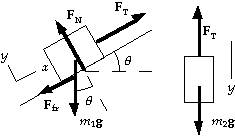```
27.	The direction of the kinetic friction force is determined by
the direction of the velocity, not the direction of the
acceleration.  We assume the block on the plane is moving up,
so the friction force is down.  The forces and coordinate systems
are shown in the diagram.  From the force diagram, with
the block m2 as the system, we can write Sum(F) = ma:
y-component: 	m2g - FT = m2a.
From the force diagram, with the block m1 as the system,
we can write Sum(F) = ma:
x-component: 	FT - Ffr - m1g sinq  = m1a;
y-component:	FN - m1g cosq = 0; with  Ffr =  ukFN.
When we eliminate FT between these two equations, we get
a  	= (m2 - m1 sinq - ukm1 cosq)g/(m1 + m2).
(a)	For a mass m1 = 5.0 kg, we have
a  	= [5.0 kg - (5.0 kg) sin 30o - (0.10)(5.0 kg) cos 30o](9.80 m/s2)/(5.0 kg + 5.0 kg)
=         2.0 m/s2 up the plane.
The acceleration is up the plane because the answer is positive.  This agrees with our assumption
for the direction of motion.
(b)	For a mass m1 = 2.0 kg, we have
a  	= [5.0 kg - (2.0 kg) sin 30o - (0.10)(2.0 kg) cos 30o](9.80 m/s2)/(2.0 kg + 5.0 kg)
=         5.4 m/s2 up the plane.
The acceleration is up the plane because the answer is positive.  This agrees with our assumption
for the direction of motion.
```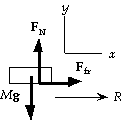```41.	The static friction force provides the centripetal acceleration.
We write Sum(F) = ma from the force diagram for the coin:
x-component:  Ffr = mv2/R;
y-component:  FN - mg = 0.
The highest speed without sliding requires  Ffr,max = usFN.
The maximum speed before sliding is
vmax = 2 pi R/Tmin = 2 pi Rfmax
= 2 pi (0.120 m)(50 /min)/(60 s/min) = 0.628 m/s.
Thus we have
usmg = mvmax2/R
us(9.80 m/s2) = (0.628 m/s)2 /(0.120 m), which gives us =       0.34.
```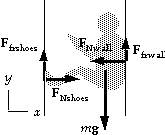```84.	We assume there is no tension in the rope and simplify the
forces to those shown.  From the force diagram, we have Sum(F) = ma:
x-component:	FNshoes - FNwall = 0,
so the two normal forces are equal: FNshoes = FNwall = FN;
y-component:	Ffrshoes + Ffrwall -  mg = 0.
For a static friction force, we know that Fsfr ~ usFN.
The minimum normal force will be exerted when the static
friction forces are at the limit:
usshoesFNshoes + uswallFNwall = mg;
(0.80 + 0.60)FN = (70 kg)(9.80 m/s2), which gives      FN = 4.9 x 102 N.

``````86.	(a)	If motion is just about to begin, the static friction
force on the block will be maximum: Ffr,max = usFN ,
and the acceleration will be zero.  We write
Sum(F) = ma from the force diagram for each object:
x-component (block):   FT - usFN = 0;
y-component (block):   FN - m1g = 0;
y-component (bucket):  (m2 + msand)g - FT = 0.
When we combine these equations, we get
(m2 + msand)g = usm1g, which gives
msand 	= usm1 - m2 = (0.450)(28.0 kg) - 1.00 kg
=      11.6 kg.
(b)	When the system starts moving, the friction becomes kinetic,
and the tension changes.  The force equations become
x-component (block):   FT2 - ukFN = m1a;
y-component (block):   FN - m1g = 0;
y-component (bucket):  (m2 + msand)g - FT2 = (m2 + msand)a.
When we combine these equations, we get
(m2 + msand - ukm1)g = (m1 + m2 + msand)a, which gives
a = [1.00 kg + 11.6 kg - (0.320)(28.0 kg)](9.80 m/s2)/(28.0 kg + 1.00 kg + 11.6 kg) =       0.879 m/s2.
```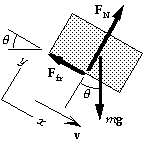```18.	(a)	While the block is sliding down, friction will be up the
plane, opposing the motion.  From the force diagram for
the block, we have Sum(F) = ma:
x-component:	mg sinq - ukFN = ma.
y-component:	FN - mg cosq = 0.
When we combine these, we have
a 	= g(sinq - uk cosq)
= (9.80 m/s2)[sin 22.0o - (0.17) cos 22.0o] =       2.1 m/s2.
(b)	For the motion of the block, we have
v2 = v02 + 2a(x - x0) = 0 + 2(2.13 m/s2)(9.3 m),
which gives
v =       6.3 m/s.
```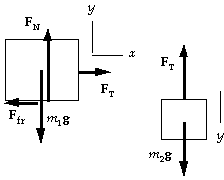```24.	(a)	For the hanging box we can write Sum(F) = ma:
y-component:  m2g - FT = 0.
For the box on the table we can write Sum(F) = ma:
x-component: FT - Ffr = 0;
y-component:  FN - m1g = 0.
When we combine the equations, we have
Ffr = FT  =  m2g = usFN = usm1g.
Thus we have
m1 - m2/us = (2.0 kg)/(0.40) =      5.0 kg.
(b)	The acceleration is zero, so the only change is that
the friction is kinetic:
m1 - m2/uk = (2.0 kg)/(0.30) =      6.7 kg.
```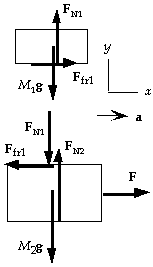```32.	(a)	The two blocks must have the same acceleration.
From the force diagram for the top block we have
x-component:   Ffr1 = M1a;
y-component:   FN1 - M1g = 0,  or  FN1 = M1g.
For the static friction force we have
Ffr1 = M1a = usFN1 = usM1g.
Thus we have
us = a/g = (5.2 m/s2)/(9.80 m/s2) =      0.53.
(b)	If the coefficient of friction is less, the top block will slide.
Because the friction force is one-half the force in part (a),
the acceleration is also reduced by half:
a1 = 1/2a = 1/2(5.2 m/s2) =      2.6 m/s2.
(c)	For the relative acceleration we have
a12 = a1 - a = 2.6 m/s2 - 5.2 m/s2 =      - 2.6 m/s2.
(d)	From the force diagram for the bottom block we have
x-component:   F - Ffr1 = M2a;
y-component:   FN2 - FN1 - M2g = 0,  or  FN2= M1g + M2g.
For part (a), if we add the two x-equations, we get
Fa 	= M1a + M2a
= (4.0 kg + 12.0 kg)(5.2 m/s2) =      83 N.
For part (b), if we add the two x-equations, we get
Fb 	= M1a1 + M2a
= (4.0 kg)(2.6 m/s2) + (12.0 kg)(5.2 m/s2) =      73 N.
```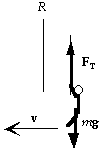```44.	The net force on Tarzan will provide his centripetal acceleration,
which we take as the positive direction.
We write Sum(F) = ma from the force diagram for Tarzan:
FT - mg = ma = mv2/R.
The maximum speed will require the maximum tension that Tarzan can create:
1400 N - (80 kg)(9.80 m/s2) = (80 kg)v2/(4.8 m), which gives v =      6.1 m/s.
``````74.	We write Sum(F) = ma from the force diagram for the stationary
hanging mass, with down positive:
mg - FT = ma = 0;   which gives
FT = mg.
For the rotating puck, the tension provides the centripetal
acceleration, Sum(FR) = MaR:
FT = Mv2/R.
When we combine the two equations, we have
Mv2/R = mg, which gives  v = (mgR/M)1/2.
```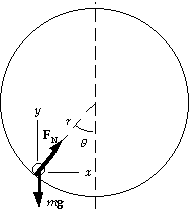```92.	(a)	If the bead does not move along the hoop, it must have only
the radial acceleration moving in a circle of radius R = r sinq.
Since f = 1/t, then v = 2 pi R f = 2 pi r sinq f.
We write Sum(F) = ma from the force diagram:
x-component: 	FN sinq = maR = mv2/R;
FN sinq = mR(2pi f)2 = 4pi2f2mr sinq;
y-component: 	FN cosq - mg = 0,  or  FN = mg /cosq.
Combining these, we get
cosq = g/4pi2f2r.
(b)	For the given data we get
cosq = (9.80 m/s2)/4pi2(4.0 rev/s)2(0.20 m) = 0.0776,
q =     86o.
(c)	Because there is no friction, the bead      cannot      ride as high
as the center.  There would be no force to balance the downward
force of gravity.

```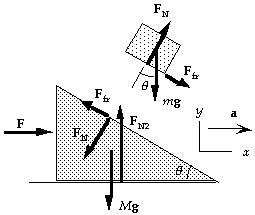```33.	If the triangular block is pushed so that the small block
tends to move up the incline, the static friction force on
the small block will be down the incline, as shown.
We choose a coordinate system with the x-axis in the
direction of the acceleration a of the triangular block.
From the force diagram for the small block we have
x-component:	FN sinq + Ffr cosq = max;
y-component:	FN cosq - mg - Ffr sinq = may.
The top block will not slide until Ffr > mFN.  As long as
this is not true, ax = a, and ay = 0.  Thus we find the
limiting acceleration by using these conditions:
FN cosq - mg - mFN sinq = 0,  or  FN = mg/(cosq - m sinq);
FN sinq + mFN cosq = mamax ,  or
amax = FN(sinq + m cosq)/m = g(sinq + m cosq)/(cosq - m sinq).
From the force diagram for the triangular block we have
x-component:	F - FN sinq - Ffr cosq = Ma.
Thus the maximum force F for the small block to not slide, and thus the minimum force to make the small block slide, is
Fmin = FN sinq + Ffr cosq + Mamax = (m + M)amax
=       (m + M)g(sinq + m cosq)/(cosq - us sinq).
```